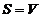§ 2   Linear spaces and linear subspaces

1.  Linear space

[ Linear operations ]    Let F be a field whose elements a , b , c , ... as quantities; V be a set of objects of any kind whose elements are denoted by the Greek letters α , β , γ , ... . Determine the two algorithms : Addition of elements in 1
o V. For
any two elements α and β in V , there is always a unique definite element γ corresponding to them, which is called the sum of α and β , denoted as . 2 o The number in F is the same as that in V Element-wise multiplication . For any number a in F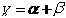With any element α in V , there is always a unique and definite element δ corresponding to them, which is called the multiplication of a and α , and is written as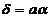These two algorithms are called linear operations .
[
Linear space and its properties ]    Let F be a field and V be a set of objects of any kind. If the following conditions are satisfied for linear operations, then V is called a linear space on the field F : ( i) V is an additive group; ( ii) For any element aF and αV , corresponding to a uniquely determined element ( iii) satisfies the distributive law and the associative law, that is, the element with field F is called The number of linear spaces, the element of V is called its vector, so the linear space is also called the vector space . The identity element of the additive group is called the zero vector, denoted as 0 , ( -1 ) α is the inverse of αV , called is a negative vector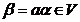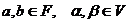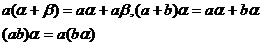A

linear space over the real number field is called a real linear space; a linear space over the complex number field is called a complex linear space .

Example 1   All the vectors in the three-dimensional space form a real linear space .

Example 2   The polynomial ring F [ x ] on the number field F forms a linear space on the number field F according to the usual polynomial addition and polynomial multiplication .

Example 3   An m × n matrix whose elements belong to the number field F form a linear space on the number field F according to the addition of the matrix and the multiplication of the matrix and the number .

Example 4   According to usual addition and multiplication, the ensemble of real numbers is a linear space over the field R of real numbers . The ensemble of complex numbers is a linear space over the field C of complex numbers. Any field is a linear space that uses itself as the field of quantities .

Example 5   takes each continuous real function defined in a real interval ( a , b ) as an element, and the sum of any two elements f and g is denoted as a continuous real function defined in The value of a point x is specified as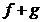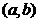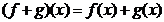The element obtained by multiplying an element f by a real number c is defined as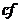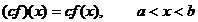Then all these elements form a real linear space .

Linear spaces have the following properties:

1 o zero vector is unique .

2o Negative vectors are unique .

3o . _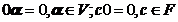4 o If then c = 0 or α = 0 .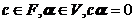[ Linearly dependent and linearly independent ]   A finite set of vectors in a linear space V over the field F , if true , only then the equation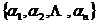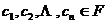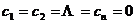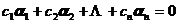The vector group is called linearly independent, otherwise it is called linearly dependent . If the vector group is linearly dependent, at least one of the vectors is a linear combination of the remaining vectors :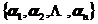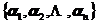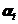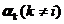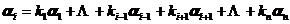Any set of vectors containing the zero vector 0 is linearly dependent .

Assuming that convergence is defined on the linear space V over the field F (Chapter 21, § 3 , 4) , a set of infinitely many vectors in V , A vector is said to be linearly independent if the time - only equation in F holds, otherwise it is said to be linearly dependent .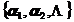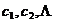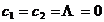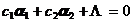[ Basis and coordinates ]   A set of vectors in the linear space V on the field F if the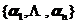(i) are linearly independent ;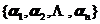(ii) Any vector in V is a finite linear combination of vectors; then it is called a finite basis of V , also called generating (or expanding) this space, which is a set of generators of the space .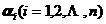Set as a set of bases of V , then any vector α in V must be represented by a linear combination: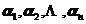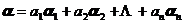The complex number in the formula is uniquely determined, and it is called the coordinate of the vector α with respect to the base .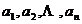If V has a finite basis, it is called a finite-dimensional linear space, otherwise, it is called an infinite-dimensional space . The number of vectors of the basis of a finite-dimensional linear space V is called the dimension of V, denoted by .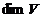[ First Dimension Theorem ]   Any two bases of a finite-dimensional linear space V over a field F have the same number of elements .

The inference is assumed to be a set of linearly independent vectors in an n -dimensional linear space V. Obviously , there is a basis in V that is a part of it .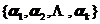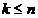2.  Linear subspace

[ Linear subspace ]   Let S be a non-empty subset of the linear space V on the field F. If the linear operation of S on V also constitutes a linear space, then S is called a linear subspace of V , which is referred to as a subspace for short .

Let S be a subset of the linear space V over the field F , if closed with respect to linear operations, that is

(i) if then ;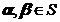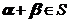(ii) if , then ;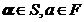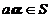Then S is a subspace of V.

For example, a subset of a single zero vector in a linear space V is a subspace of V , called the zero subspace . V itself is also a subspace of V. These two subspaces are called trivial subspaces of V.

Let be a set of vectors in the linear space V on the field F , all linear combinations of this set of vectors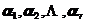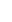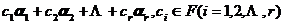A subspace that constitutes V is called the subspace generated (or stretched) by . This is a non-trivial subspace of V.[ Intersection and Sum of Subspaces ]   Let S , T be the subspace of the linear space V on the field F , and the subset formed by all the vectors in V belonging to S and T is called the intersection (communication set) of S and T , Denoted as . The subset composed of all vectors that can be represented as S and T is called the sum (sum set), denoted as (or ) .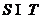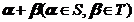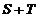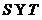Let S and T be two subspaces of a linear space V on F , then the intersection and sum of S and T are both subspaces of V.[ Second dimension theorem ]   Let S and T be two subspaces of linear space V , then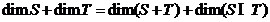(here denotes the dimension of the linear space V ) .It can be deduced that if the sum of the dimensions of the two subspaces S and T in the n -dimensional linear space V is greater than n , then S and T must contain a common non-zero vector .

For example, two different planes (two-dimensional subspaces) in three-dimensional space intersect on a straight line, because , but , so .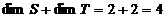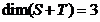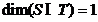[ Direct sum of subspaces ]   Let be a subspace of the linear space V , if the decomposition of each vector α in the sum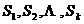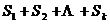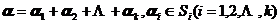is unique . This sum is called a straight sum and is written as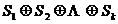Direct sums of subspaces have the following properties:

The necessary and sufficient conditions for 1o to be a direct sum are: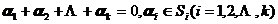This is true only for all-zero vectors .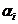The necessary and sufficient conditions for the sum of 2o to be a direct sum are: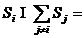Φ (empty set)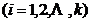3 Let o be a subspace of the linear space V , if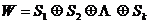but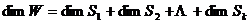The reverse is also true .

This shows that for a direct sum of subspaces, the dimension is additive . It can be seen that ifthe basis of the subspace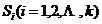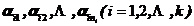Combined, we get a set of bases for the subspace W.

[ quotient space ]   Let S be a subspace of V , and set two vectors , if , then the sum is equivalent, denoted as . In fact, this relation has three properties of equivalence relation: ( i) Reflexive For each , there are ; ( ii ) symmetry if , then ; ( iii) transitivity if , , then . As in the case of sets, two equivalent vector sums are said to belong to the same class . Each vector contains exactly In a class, this class is denoted . The zero vector 0 in V is contained in the class coincident with the subspace S.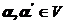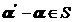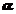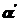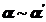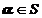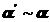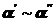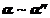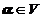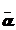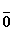If each class is taken as an element, then the set of all these elements is a linear space, called the quotient space of V with respect to S , denoted as . The zero vector of the quotient space is , and has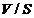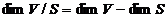It can be seen from this that if , the dimension of the quotient space is zero; and if S is a null space, the dimension of the quotient space is the same as that of V.